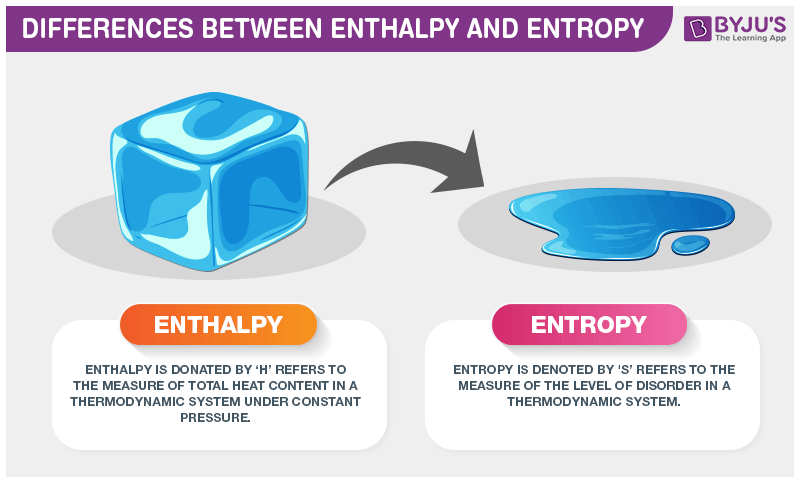# Difference Between Enthalpy and Entropy

We know that the major difference between enthalpy and entropy is that even though they are part of a thermodynamic system, enthalpy is represented as the total heat content whereas entropy is the degree of disorder.In a closed system,

$$\begin{array}{l}T.\Delta S=\Delta H\end{array}$$
, which means that in absolute temperature with some change in entropy results in a change of enthalpy.

## Difference Between Enthalpy and Entropy

Enthalpy is the measure of total heat present in the thermodynamic system where the pressure is constant.

It is represented as

$$\begin{array}{l}\Delta H=\Delta E+P\Delta V\end{array}$$
where E is the internal energy.

Entropy is the measure of disorder in a thermodynamic system. It is represented as

$$\begin{array}{l}\Delta S=\Delta Q/T\end{array}$$
where Q is the heat content and T is the temperature.

Difference Between Enthalpy and Entropy
Enthalpy Entropy
Enthalpy is a kind of energy Entropy is a property
It is the sum of internal energy and flows energy It is the measurement of the randomness of molecules
It is denoted by symbol H It is denoted by symbol S
It was termed by a scientist named Heike Kamerlingh Onnes It was termed by a scientist named Rudolf Clausius
It unit is
$$\begin{array}{l}Jmol^{-1}\end{array}$$
It unit is
$$\begin{array}{l}JK^{-1}\end{array}$$
It related is applicable in standard conditions It does not have any limits or conditions.
The system favor minimum enthalpy The system favor maximum entropy

These were some difference between Enthalpy and Entropy. If you wish to find out more, download BYJU’S The Learning App.

RELATED ARTICLES:

Test your knowledge on Enthalpy and entropy differences